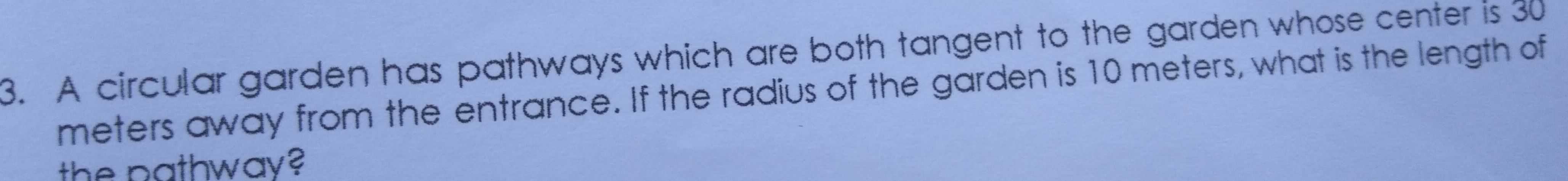### ¿Todavía tienes preguntas de matemáticas?

Pregunte a nuestros tutores expertos
Algebra
Pregunta3. A circular garden has pathways which are both tangent to the garden whose center is $$30$$ meters away from the entrance. If the radius of the garden is $$10$$ meters, what is the length of the oathway?

$$L = \sqrt{30^2 - 10^2} = \sqrt{800} \\L = 28.28 \space m$$